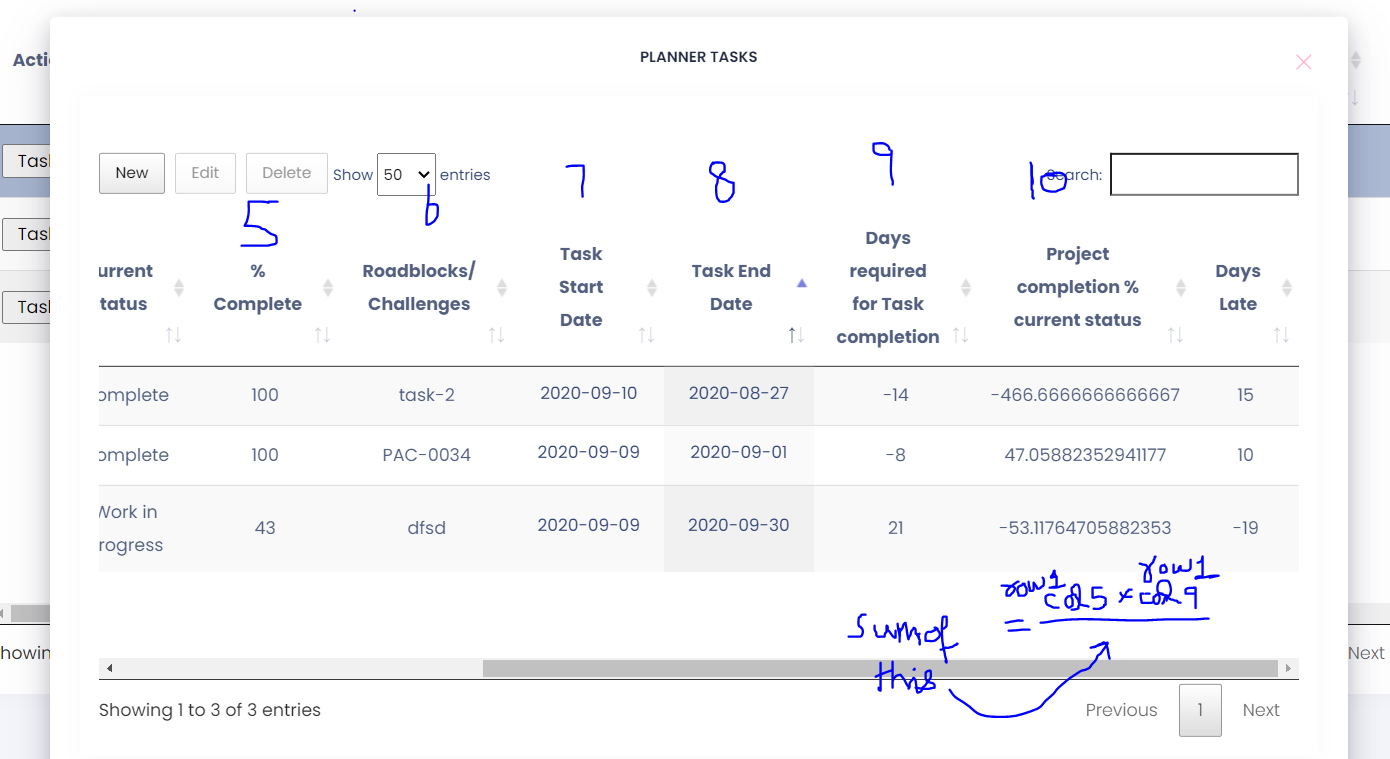# Need sum of column into next columns' of every row

edited September 11

I need the sum-of-column (9) in the next column (10). In column(10), I have to implement given formula like this;
row1.column(5) x row1.column(9) / SumOf(allRows.column(9))
but I don't know how to implement this logic in datatable.
because if the user creates a new row then this new row's column(9) value should add into other rows' column(9) like in the formula above mentioned.
I searched and found footerCallback but unfortunately, it wasn't the solution to my problem I'm looking for.

``````  **column-7**{
data: "TaskStartDate", render: function (data) {
var returnVal = dateFormat(data);
return '<label>' + returnVal + '</label>';
}
},
**column-8**{
data: "TaskEndDate", render: function (data) {
var returnVal = dateFormat(data);
return '<label class="date-width">' + returnVal + '</label>';
}
},
**column-9**{
data: null, render: function (data, type, row, meta) {
var daysRequired = countRequiredDays(start, end);
daysRequiredForTaskComplete = daysRequired;// need this value for next column calculation.
return daysRequired;
}
},
**column-10 **  {
data: null, render: function (data) {
return completionPercentageCurrentStatus;
}
}
``````

Note that:
column 9 and 10 both are my auto-generated formula column. I'm not saving these values into the database as I don't need them in my database. And in column-9 I'm getting values from column-7 and column-8.Edited by Colin - Syntax highlighting. Details on how to highlight code using markdown can be found in this guide.

You could either do that in `columns.render`, where the third parameter is the data for all the row, or you could do it `createdRow`,

Colin

• Hi @colin !
this is a rough-live example link:
http://live.datatables.net/behomisa/1/edit
would you help me to show how to implement that logic I've mentioned above?

for example according to the attached live-example. I need a column named "Total".
in this "Total" column I need calculated values of row[nth].stock * row[nth].Nb / sumof (Column(Nb)).
so the issue I'm facing is that when I try to add a new row then sumOf(column(Nb)) will be changed too and affect the calculated values in the "Total" column.

if you want I can share a TeamViewer access with you just let me know a day and a time, and where you're from?

I need to implement this logic its a client requirement. I will be very grateful for your support.